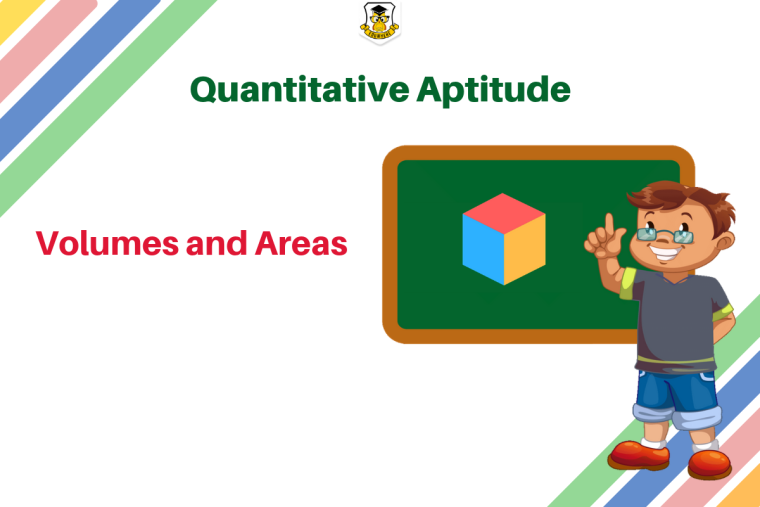Eduwhere's Concept: Volumes and AreasIn Mensuration, it is essential to be well versed with the formulae of the geometrical figures.  Candidates can face questions related to the same in the Quantitative Aptitude section of their upcoming competitive examination.

Take a quick look at the important formulae for volumes and areas.

Square:

Area = S²

Perimeter = 4s

s = length of the sides, d = length of diagonal.

Triangle

Area = ½ x base x height

Perimeter = x + y + z ( summation of three sides of a triangle)

Rectangle

Area = base x height = b x h

Perimeter = 2 (b + h)

Rhombus

Area = ½ x product of the diagonals between the sides * sine of the angle between the sides

Perimeter = 4 x side (any side)

Diagonal = 2 x area / diagonal

Trapezium

Area = ½ × sum of the parallel sides × height.

Parallelogram

Area = product of any two sides x sine of the included angle

Perimeter = 2 (a + b) (a and b are the two adjacent sides)

Volumes of various solids:

Cube

In a cube, length = breadth = height = s

The surface area of a cube = 6s²

The volume of a cube = S³

Diagonal of a cube = √3 s

Cylinder

The curved surface area of a cylinder: 2 πrh( r = radius of the base, h = height)

The total surface area of a right circular cylinder = 2 πrh + 2 πr²

The volume of the right circular cylinder: πr²h

Sphere

The surface area of a sphere: 4 πr²

The volume of a sphere: 4/3 πr³

A half-sphere is known as a hemisphere.

Cone

The curved surface area of a cone: pirl (l is the slant height)

The total surface area of a cone: πrl + πr²

The volume of a cone: 1/3 πr²h

Cuboid

Total surface area of a cuboid: 2 (lb + bh + lh)

The volume of a cuboid: lbh

Hemisphere

The curved surface area of a hemisphere: 3 πr2

The total surface area of a hemisphere: 3 πr2

The volume of a hemisphere: 2/3 πr3

2

1457